# I.E. Irodov Solutions on The Second Law of Thermodynamics- Entropy

The second law of thermodynamics that provides the criterion for the feasibility of any process. According to the second law of thermodynamics, "for any spontaneous process the entropy of the universe increases". The second law of thermodynamics put restrictions upon the direction of heat transfer and achievable efficiencies of heat engines. Subject experts have provided a detailed explanation of each question asked in I.E. Irodov book PART TWO- THERMODYNAMICS AND MOLECULAR PHYSICS - 2.4- The Second Law of Thermodynamics- Entropy. By referring to these solutions, students can achieve higher marks in the JEE exam. Students are recommended to go through these solutions so that they can be familiar with the type of questions from The Second Law of Thermodynamics.

### I.E. Irodov Solutions on The Second Law of Thermodynamics- Entropy

1. In which case will the efficiency of a Carnot cycle be higher: when the hot body temperature is increased by ΔT, or when the cold body temperature is decreased by the same magnitude?

Solution:

1. The efficiency is given by

η = (T1-T2)/T1, (T1 > T2)

Now in the two cases the efficiencies are

$\eta_k = \frac{T_1+\Delta T-T_2}{T_1+\Delta T}, T_1 \ increased \ and \ \eta_l = \frac{T_1+\Delta T-T_2}{T_1}, T_2 \ decreased$

Thus, ηk < ηl

2. Hydrogen is used in a Carnot cycle as a working substance. Find the efficiency of the cycle, if as a result of an adiabatic expansion (a) the gas volume increases n = 2.0 times; (b) the pressure decreases n = 2.0 times.

Solution:

1.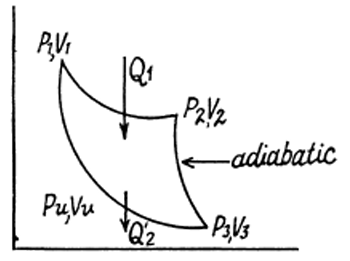For H2, γ = 7/5

p1V1 = p2V2 , P3V3 = p4V4

p2V2γ = p3V3 γ, P1V1 γ = p4V4 γ

Define n by V3 = nV2

Then p3 = p2 n- γ, so

p4V4 = p3V3 = p2V2 n 1-γ = p1V1 n 1-γ

p4V4 γ= p1V1γ

so, V41-γ = V11-γ n 1-γ

or V4 = nV1

Also,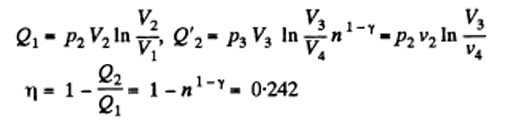(b) Define n by p3 = p2/n

p2V2γ = (p2/n)V3γ or V3 = n1/γ V2

If n approaches to n1/γ in the previous case

Η = 1-n1/γ-1 = 1 - n-2/7 = 0.18(approx.)

3. A heat engine employing a Carnot cycle with an efficiency of η = 10% is used as a refrigerating machine, the thermal reservoirs being the same. Find its refrigerating efficiency ε.

Solution:

1.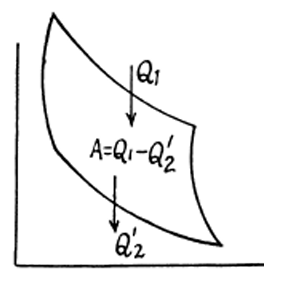Used as a refrigerator, the refrigerating efficiency of a heat engine is given by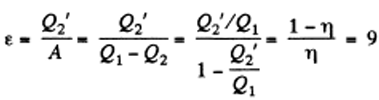where η is the efficiency of the heat engine.

4. An ideal gas goes through a cycle consisting of alternate isothermal and adiabatic curves (below Fig.). The isothermal processes proceed at the temperatures T1, T2, T3. Find the efficiency of such a cycle, if in each isothermal expansion the gas volume increases in the same proportion.

Solution:

1.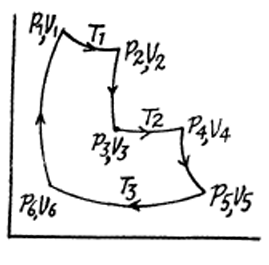Given: V2 = nV1, V4 = nV3

Q1 = Heat taken at the upper temperature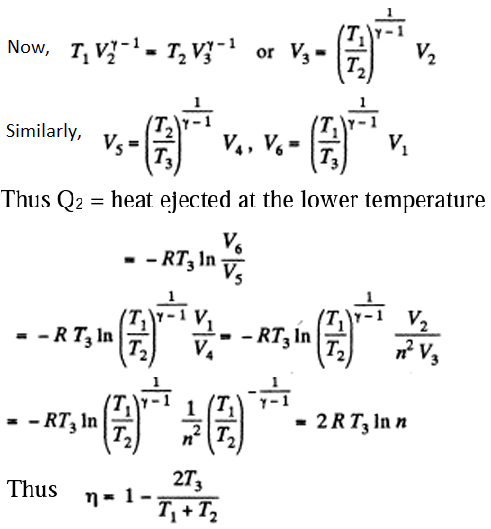5. Find the efficiency of a cycle consisting of two isochoric and two adiabatic lines, if the volume of the ideal gas changes n = 10 times within the cycle. The working substance is nitrogen.

Solution:

1.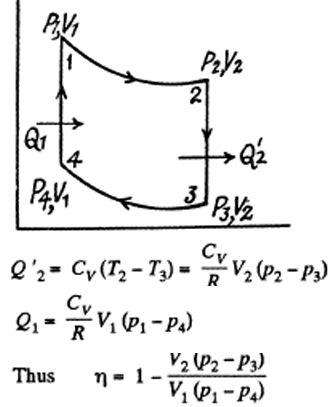on the other hand,

6p1V1γ = p2V2γ, p3V2γ = p4V1γ

Also, V2 = nV1

Thus, p1 = p2nγ , p4 = p3nγ

η = 1 – n1-γ with γ = 7/5 for N2.

6. Find the efficiency of a cycle consisting of two isobaric and two adiabatic lines, if the pressure changes n times within the cycle. The working substance is an ideal gas whose adiabatic exponent is equal to γ.

Solution:

1.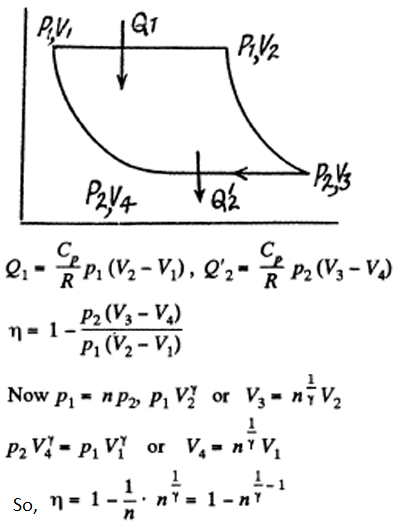7. An ideal gas whose adiabatic exponent equals γ goes through a cycle consisting of two isochoric and two isobaric lines. Find the efficiency of such a cycle, if the absolute temperature of the gas rises n times both in the isochoric heating and in the isobaric expansion.

Solution:

1. Since the absolute temperature of the gas rises n times both in the isochoric heating and in the isobaric expansion.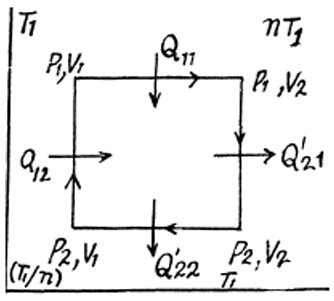p1np2 and V2 = nV1

Heat taken is Q1 = Q11 + Q12

Q11 = cp(n-1)T1 and Q12 = cv(1-1/n)T1

Heat rejected is Q' 2 = Q' 21 + Q’22 where

Q21 = cv(n-1)T1 and Q22 = cp(1-1/n)T1

Thus,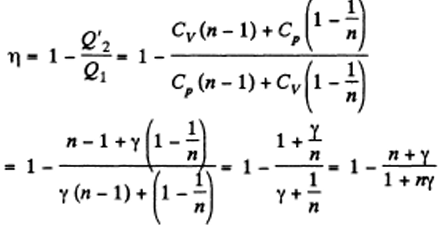8. An ideal gas goes through a cycle consisting of (a) isochoric, adiabatic, and isothermal lines; (b) isobaric, adiabatic, and isothermal lines, with the isothermal process proceeding at the minimum temperature of the whole cycle. Find the efficiency of each cycle if the absolute temperature varies n-fold within the cycle.

Solution:

1.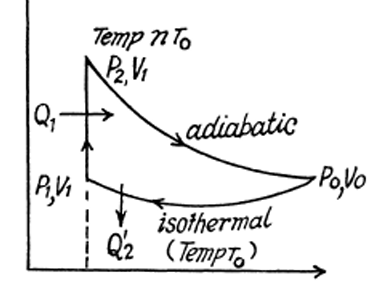(a) p2 = np1, p1V1 = p0V0,

np1V1γ = p0V0 γ

Q’2 = RT0 ln(Vo/V1), Q1 = CvTo(n-1)

But nV1γ-1 = V0 γ-1 or V1 = V0n-1/(γ-1)

Q’2 = RT0 ln(n1/(γ-1)) = [RTo/(γ-1)] ln n

Thus, η = 1 – ln n/(n-1) on using Cv = R/(γ-1)

(b) V2 = nV1, p1V1 = p0V0,

And p1 (nV1) γ = p0V0 γ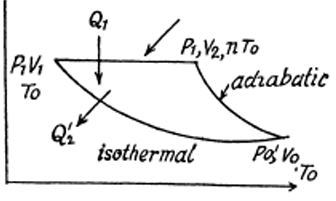i.e. nγ V1 γ-1 = V0 γ-1 or V1 = n- γ/( γ-1 V0

Also, Q1 = CpT0(n-1), Q’2 = RT0 ln(Vo/V1)

Or Q’2 = RT0 ln(n γ/(γ-1)) = Rγ/(γ-1) To ln n = Cp To ln n

Thus, η = 1 – (ln n)/(n-1)

9. The conditions are the same as in the foregoing problem with the exception that the isothermal process proceeds at the maximum temperature of the whole cycle.

Solution:

1. Solution: Here the isothermal process proceeds at the maximum temperature instead of at the minimum temperature of the cycle.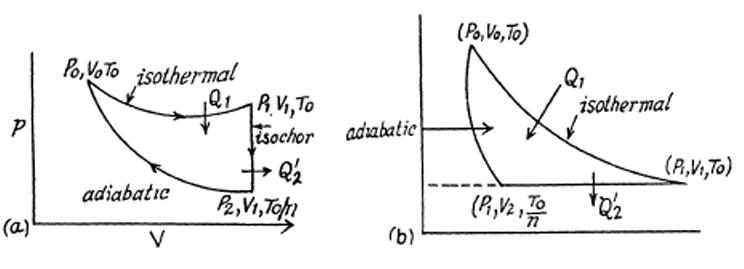(a) p2 = p1/n, p1V1 = p0V0

p2V1γ = p0V0 γ or p1V1γ = np0V0 γ

i.e. V1γ-1 = nV0γ-1 or V1 = V0n1/(γ-1)

Q’2 = CvT0 (1-1/n), Q1 = RT0 ln(V1/V0) = [RTo/(γ-1)] ln n = CvTo ln n.

Thus, η = 1 – Q’2/Q1 = 1 – (n-1)/(n ln n)

(b) p2 = V1/n, p1V1 = p0V0

P0Voγ = p1V2 γ = p1 n V1γ = V0γ-1 n V1γ-1 or V1 =n (γ /(γ-1) V0

Q’2 = CpT0 (1-1/n), Q1 = RT0 ln(V1/V0) = [R γ/(γ-1)] To ln n = CpTo ln n.

Thus, η = 1 – Q’2/Q1 = 1 – (n-1)/(n ln n)

10. An ideal gas goes through a cycle consisting of isothermal, polytrophic, and adiabatic lines, with the isothermal process proceeding at the maximum temperature of the whole cycle. Find the efficiency of such a cycle if the absolute temperature varies n-fold within the cycle.

Solution:

1. The section from (p1, V1, T0) to (p2, V2, T0/n) is a polytrophic process of index a. We shall assume that the corresponding specific heat C is + ve.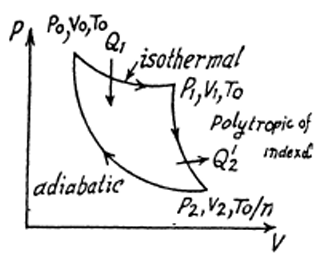Here dQ = CdT = CvdT + pdV

Now, pVα = constant or TVα-1 = constant

So, pdV = (RT/V)dV = -(R/(α-1))dT

Then $C = C_v - \frac{R}{\alpha -1} = R(\frac{1}{\gamma -1} - \frac{1}{\alpha -1})$

We have, p1V1 = RT0 = p2V2 = RTo/n = p1V1/n

p0V0 = p1V1 = n p2V2, p0V0γ= p2V2 γ,

p1V1α = p2V2 α or V0γ-1 = (1/n)V2 γ-1 or V2= Vo (n)1/(γ-1)

V1α-1 = (1/n)V2 α-1 or V1 = n(-1/ α-1), V2 = (n)1/(γ-1) – 1/( α-1) V0

Now, Q’2 = CT0(1 – 1/n), Q1 = RTo ln(V1/V0) = RTo(1/(γ-1) – 1/(α-1)ln n = CT0 ln n

Thus, η = 1 – Q’2/Q1 = 1 – (n-1)/(n ln n)

11. An ideal gas with the adiabatic exponent γ goes through a direct (clockwise) cycle consisting of adiabatic, isobaric, and isochoric lines. Find the efficiency of the cycle if in the adiabatic process the volume of the ideal gas (a) increases n-fold; (b) decreases n-fold.

Solution:

1.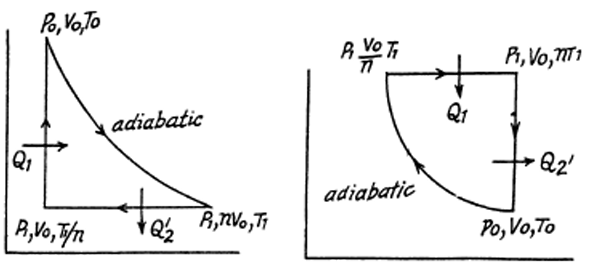(a) Here $Q_2' = C_p(T_1-T_1/n) = C_pT_1(1-1/n), \ Q_1=C_v(T_0-T_1/n)$

Along the adiabatic line, ToVoγ-1 = T1(nVo) γ-1 or To = T1n γ-1

So, Q1 = Cv(T1/n)(nγ -1)

Thus, η = 1 – [γ(n-1)]/[ (nγ -1)]

(b) Here $Q_2' = C_v(nT_1-T_0), \ Q_1=C_pT_1(n-1)$

Along the adiabatic line, TV γ-1 = constant

ToVoγ-1 = T1(Vo/n) γ-1 or T1 = T0n γ-1

Thus, η = 1 – [nγ -1]/[(n-1)γnγ -1]

12. Calculate the efficiency of a cycle consisting of isothermal, isobaric, and isochoric lines, if in the isothermal process the volume of the ideal gas with the adiabatic exponent γ.

1. (a) increases n-fold (b) decreases n-fold.

Solution:

1.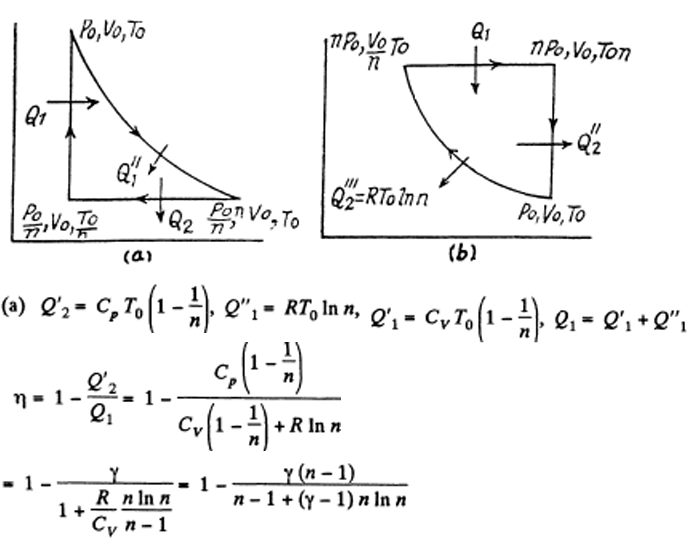(b) Here $Q_1 = C_p T_0(n-1), \ Q_2’=C_vT_0(n-1)$

Thus, η = 1 – [(n -1)+(γ-1)ln n]/[(n-1)γ]

13. Find the efficiency of a cycle consisting of two isochoric and two isothermal lines if the volume varies v-fold and the absolute temperature τ-cycle within the cycle. The working substance is an ideal gas with the adiabatic exponent γ.

Solution:

1.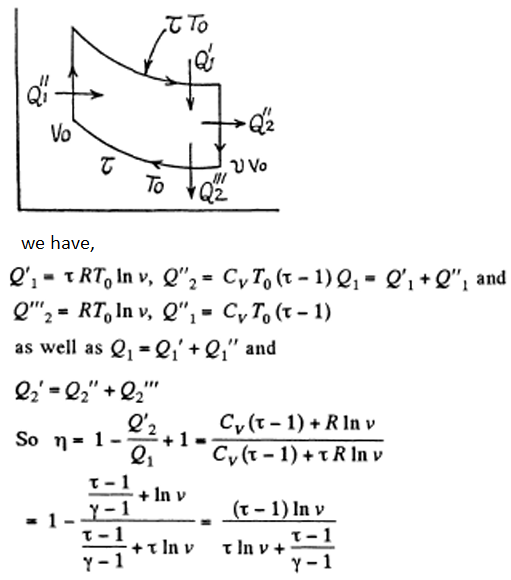14. Find the efficiency of a cycle consisting of two isobaric and two isothermal lines if the pressure varies n-fold and the absolute temperature τ-fold within the cycle. The working substance is an ideal gas with the adiabatic exponent γ.

Solution:

1.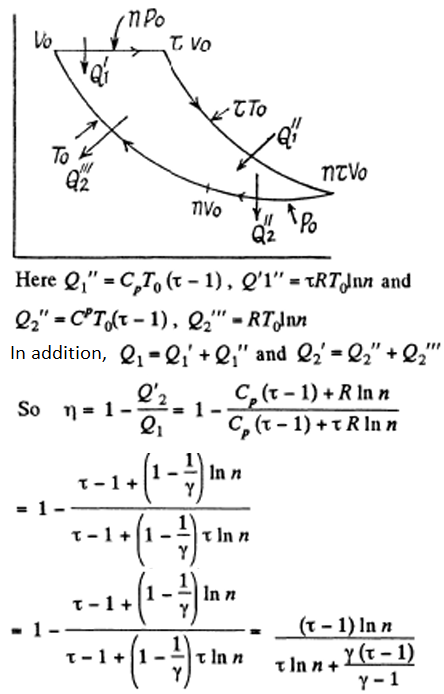15. An ideal gas with the adiabatic exponent γ goes through a cycle (Fig. below) within which the absolute temperature varies τ-fold. Find the efficiency of this cycle.

Solution:

1.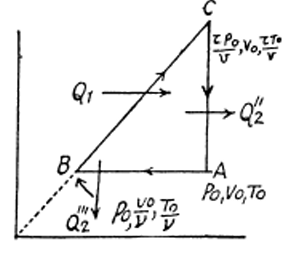Due to linearity of the section

BC whose equation is

p/po = vV/Vo

we have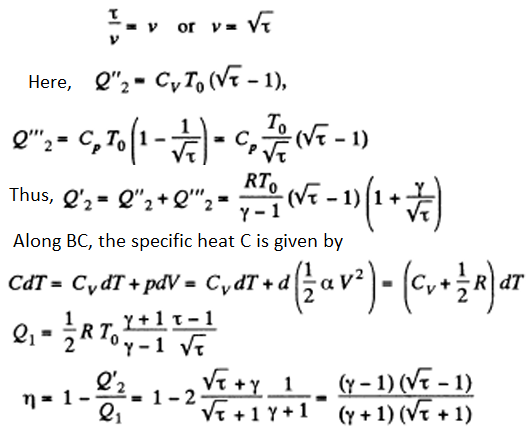16. Making use of the Clausius inequality, demonstrate that all cycles having the same maximum temperature Tmax and the same minimum temperature Tmin are less efficient compared to the Carnot cycle with the same Tmax and Tmin.

Solution:

1. Claussius inequality can be written in the form: $\int \frac{d_1 Q}{T}-\int \frac{d_2 Q}{T} \leq 0$, Where dQ is the heat transferred to the system but d2Q is heat rejected by the system, both are +ve and this explains the minus sign before d2Q.

In this inequality, Tmax > T > Tmin and we can write

$\int \frac{d_1 Q}{T_{max}}-\int \frac{d_2 Q}{T_{min}} < 0$

Thus, Tmin/Tmax < Q’2/Q1

η = 1 – Q’2/Q1 < 1 - Tmin/Tmax = ηcarnot

17. Making use of the Carnot theorem, show that in the case of a physically uniform substance whose state is defined by the parameters T and V, $(\frac{\partial U}{\partial V})_T = T(\frac{\partial p}{\partial T})_v - p$

Where U (T, V) is the internal energy of the substance.

Instruction: Consider the infinitesimal Carnot cycle in the variables p, V.

Solution:

1. We consider an infinitesimal carnot cycle with isothermal process at temperatures T + dT and T. Let δA be the work done in the cycle and δQ, be the heat received at the higher temperature. Then by Carnot's theorem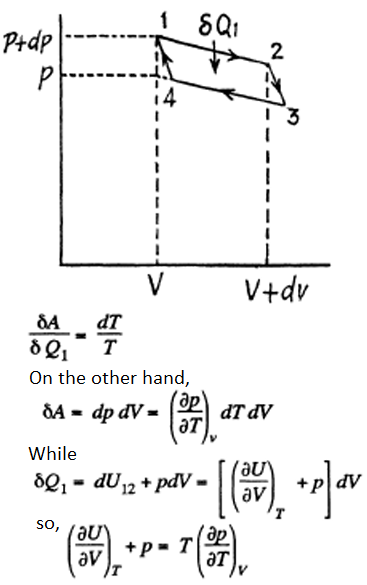18. Find the entropy increment of one mole of carbon dioxide when its absolute temperature increases n = 2.0 times if the process of heating is (a) isochoric; (b) isobaric. The gas is to be regarded as ideal.

Solution:

1. (a) In an isochoric process the entropy change will be

$\Delta S = \int_{T_i}^{T_f}\frac{C_v dT}{T} = C_v ln\ n = \frac{R\ ln\ n}{\gamma-1}$

For carbon dioxide, γ = 1.30

∆S = 19.2 Joule/oK-mole.

(b) For an isobaric process,

∆S = Cp ln (Tf/Ti ) = Cp ln n = [γR ln n]/[ γ-1] = 25 Joule/oK-mole.

19. The entropy of v = 4.0 moles of an ideal gas increases by ΔS = 23 J/K due to the isothermal expansion. How many times should the volume v = 4.0 moles of the gas be increased?

Solution:

1. In an isothermal expansion

∆S = vR ln (Vf/Vi )

Vf/Vi = e∆S/VR = 2.0 times

20. Two moles of an ideal gas are cooled isochorically and then expanded isobarically to lower the gas temperature back to the initial value. Find the entropy increment of the gas if in this process the gas pressure changed n = 3.3 times.

Solution:

1. The entropy change depends on the final & initial states only, so we can calculate it directly along the isotherm, it is ∆S = 2R ln n = 20 J/oK.

(assuming that the final volume is n times the initial volume)